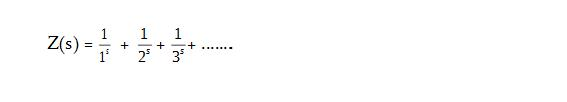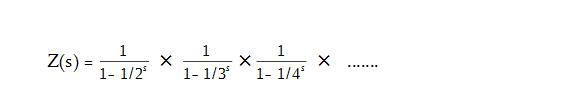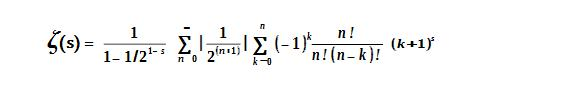# ijspace.org

 Riemann Hypothesis in j-space 4th August 2014 Updated 19th November 2018       Let us consider an infinite series Z(s) represented as,The function Z(s) also known as Euler series, is divergent for s less than or equal to 1.  The Euler series can also be written in terms of prime numbers as,Using the method of analytic continuation we can write the Riemann Zeta function as,where s can be a complex number.  We note that following properties: (i)    The Riemann Zeta function is undefined for s=1. (ii)    If RZ function does not diverge, it is equal to Z(s). (iii)   Non-trivial zeros exist for s = -2, -4, -6, ...... (iv)    For |s| < 1 (unit circle in complex-plane), non-trivial zeros exist in the complex plane such that, s = 1/2 + i r, where r is an  irrational number.     We will focus our attention on the analytic continuation first.  We note that analytic continuation allows the existence of the multi-valued functions.  In a discrete measurement space or j-space, no two measurements can be alike (hence no multi-valued functions) due to entropy associated with the finite-capability macroscopic observer ObsM.  However we can assume the measurements to be almost identical in ObsM measurement space by invoking VT symmetry.  Then the results from analytic continuation can be applied to explain observations, in terms of a series expansion in j-space.      Furthermore we note the presence of irrational numbers in the imaginary component of the non-trivial zeros.  Usually we encounter irrational numbers such as 'pi' and 'e' while describing an indeterminate phenomenon in a discrete measurement space.  Thus we have two important issues to consider, (i) the presence of a boundary at |s| = 1, or the unit circle in a complex plane, and (ii) the irrational numbers which indicate the presence of j-space, which is likely to have a quantum nature per measurements made by ObsM.     It will be worthwhile to point out a footnote (p. 526) in Adler's Quaternionic Quantum Mechanics and Quantum Fields, in which the work of Sir Michael Berry based on Odlyzko's numerical analysis of primes, is referred to.  It is indicated that the Riemann Zeros are eigenvalues of a Hamiltonian of a classical dynamical system of chaotic orbits, which is quantized with no time reversal symmetry (possibly the effect of spin is not considered).     We had discussed the observer's capability in terms of particle-in-a-box problem.  Rather than changing the complexity of the problem we consider observers with varying capabilities solving  the same problem.  For an observer of an infinite capability his measurements are described by a shallow-well.  Whereas for an observer, ObsM,  of infinitesimal capability, his measurements are described by particle-in-a-box.  The Riemann Zeros are likely to describe a system based on the measurements of ObsM.*     One of the benefits of the string theory is to establish the necessity of the abstract thought before the mathematical description is developed.  So when would a curve would cross a line or when it would not, becomes an important question to answer.  So is the question whether a sphere can ever be divided into exact eight parts? ___________ * Riemann-zeta function is also used for defining the Hamiltonian for open-strings in string theory, to calculate the critical dimensions.  The fact ζ(-1) = -1/12, combined with zero-point energy equal to 1, provides the number of  space-time dimensions equal to 26. Note: The article "A Friendly Introduction to The Riemann Hypothesis" by Thomas Wright is highly recommended along with John Derbyshire's Prime Obsession.Information on www.ijspace.org is licensed under a Creative Commons Attribution 4.0 International License.